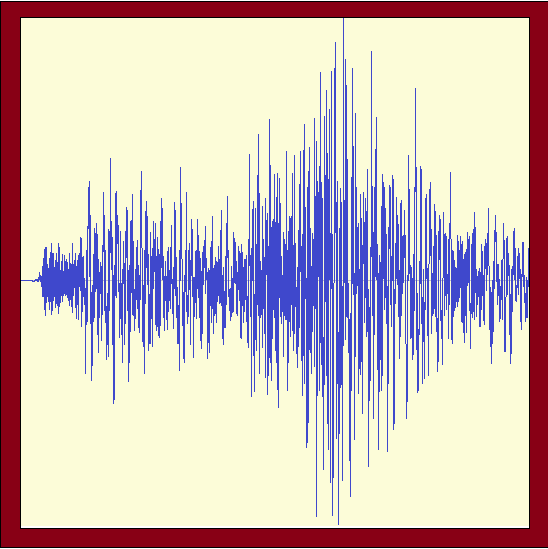# CQSRG Earthquake Recurrence Period Calculator

CQSRG (pronounced CQ Surge) has been researching the earthquake seismicity of Eastern Central Queensland since it began operation in 2002.
CQSRG is a self funded, independent, non-commercial research institute.# Gumbel Earthquake Recurrence Period Calculator

This calculator uses formulae developed by Mike Turnbull, derived from the work of Emile Gumbel, and Cinna Lomnitz. The default Alpha and Beta parameters used in this calculator are as calculated by Mike Turnbull for the Bundaberg region of Central Queensland, using historical earthquake event data available in the Geoscience Australia earthquake database from 1878 to 2005. Alpha is the average number of earthquakes in the area being considered per year above magnitude 0.0. Beta is the inverse of the average magnitude of earthquakes in the area being considered. In the case of Central Queensland the area considered was the ±3° geographical square centred on Bundaberg.

To use the calculator just change the design magnitude, the design period, or either of the Gumbel parameters. The program will recalculate the recurrence period and associated probabilities each time the values are changed.

The following important points should be noted.

• The recurrence periods calculated by this method are estimates only, based on the available data. A recurrence period of x Years means that there is a 62% probability that at least one earthquake of the stated magnitude or greater will occur within that period. It also means that there is a 38% probability that this will not occur.
• You cannot infer that as time elapses the probability that an earthquake of a particular magnitude will occur is getting greater and greater. A recurrence period of x Years means that there is a 62% probability that at least one earthquake of the stated magnitude or greater will occur within that period. The value of the recurrence period does not change from day to day! As time moves forward so does the beginning and end dates of the recurrence period!
• The calculations within the Central Queensland region are only valid for magnitudes up to about ML 6. Extrapolation beyond that is not supported by the method due to sparcity of data used to derive the alpha and beta parameters.
Gumbel Parameters
 Alpha : Beta :
Argument values
 Design magnitude (y): Design Period (T): years
Results
 Average Recurrence Period (Ty): Probability(Ty >= T) Probability(Ty < T)

# References

Turnbull M.L. & Weatherley D. 2006, Validation of using Gumbel Probability Plotting to estimate Gutenberg-Richter seismicity parameters, Proceedings of the AEES 2006 Conference, pp127 to 135, Canberra, A.C.T., November 2006, ISBN 0-646-46921-5.

Turnbull M.L. 2006, Estimation of Gutenberg-Richter seismicity parameters for the Bundaberg region using Piecewise Extended Gumbel Analysis, Proceedings of the AEES 2006 Conference, pp275 to 280, Canberra, A.C.T., November 2006, ISBN 0-646-46921-5.